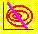5.5 MINIMUM-VARIANCE PORTFOLIO

Here we construct the portfolio that has the minimum possible risk. This will form one of the two points from which we construct the critical line.

By applying CAPM Tutor you can locate the minimum-variance portfolio graphically by first clicking on the Frontier button and then clicking on the part of the minimum-variance frontier that has the smallest possible variance. Recall, that the horizontal axis is portfolio variance and the vertical axis is portfolio return. The risk and return, associated with each point that you click on, is displayed in the data entry window.

For the mathematical problem of constructing the minimum-variance portfolio, recall that the general form is an equation of second degree: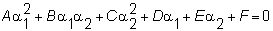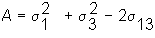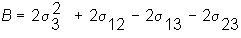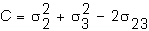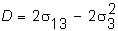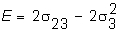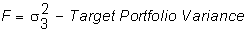The unconstrained minimum-variance portfolio is found by taking the partial derivative with respect to a1 and a2 and setting these to zero. This yields a pair of linear equations with two unknowns, which can be solved to obtain the minimum-variance portfolio weights. The weight of Security 3 is determined from setting the sum of these weights to equal one.

The two (first-order) conditions for the minimum-variance weights are: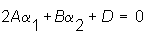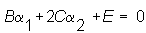The solution to this set of simultaneous linear equations is: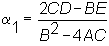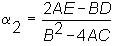The solution exists whenever the denominator is non zero.

For the Three-Firm Case, the weights for the minimum-variance portfolio are: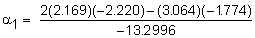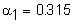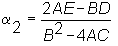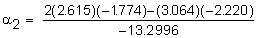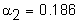and therefore the weight for Security 3 is: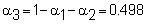By applying CAPM Tutor you can compute the minimum-variance portfolio, by first clicking on the Frontier button, and then clicking on the portfolio with the smallest variance. This allows you to see the corresponding behavior in terms of portfolio weights on the smaller graph.

The remaining part of this chapter provides a formal treatment of the construction of an isomean-isovariance tangency point, followed by a general summary of the construction of the critical line.

previous topic

next topic